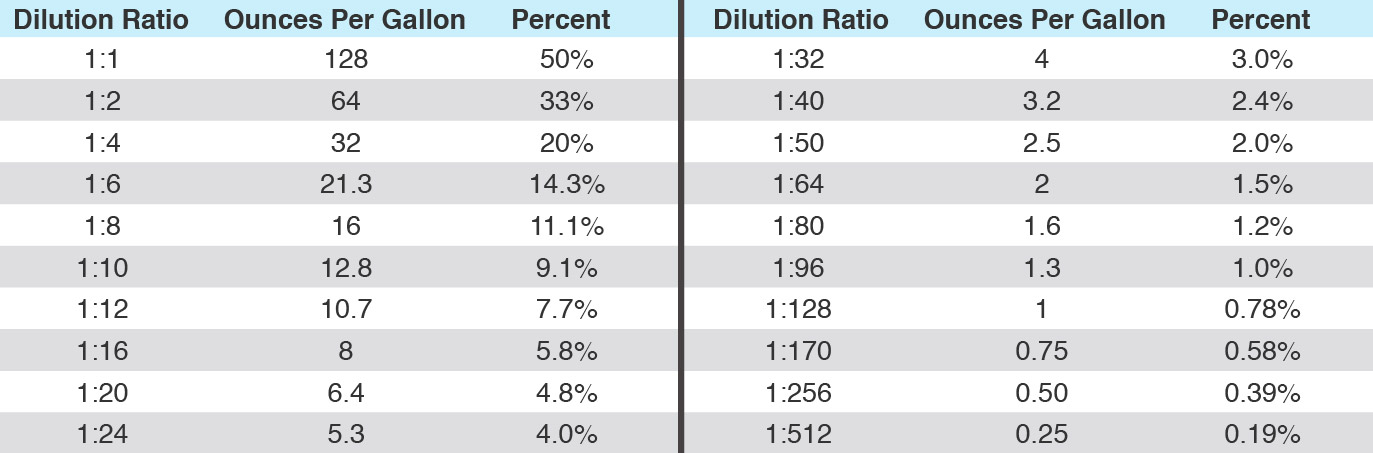Diluted Cost Per Gallon
Divide dilution into cost per gallon.
Example: (1:128 dilution selling at \$13.40 per gallon)
\$13.40 ÷ 129 = .1038 -or- 10.4¢ per diluted gallon

Dilution Ratio
Divide ounces per gallon into 128.
Example: (4 ounces per gallon dilution)
128 ÷ 4 = 32  –  Answer: 1:32 ratio

Ounces Per Gallon
Divide dilution ratio into 128.
Example: (1:32 dilution)
128 ÷ 32 = 4  –  Answer: 4 ounces per gallon

Temperature Conversion
°C = 5/9 x (°F – 32)
Example: 70°F = 5/9 x 70 – 32 = 21°C

°F = 9/5 x (°C) + 32
Example:
25°C = 9/5 x 25 + 32 = 77°F

Metric Volume Equivalents
1 fluid ounce = 30 milliliters
8 ounces (1 cup) = 237 milliliters
16 ounces (1 pint) = 473 milliliters32 ounces (1 quart) =946 milliliters
64 ounces (½ gallon) = 1892 milliliters -or- 1.9 liters
128 ounces (1 gallon) = 3785 milliliters -or- 3.8 liters

Hard Water Measurements

Hard water contains ions of magnesium and calcium.
1 grain hard water = 18 ppm hard water
Example:
450 PPM hard water = 25 grain
(450 ÷ 18 = 25)

Active Parts Per Million
Commonly used for disinfectants.
Step 1: Add together active ingredients on label.
Step 2: Multiply by 10,000.
Step 3: Divide the result of Step 2 by the dilution rate.

Example:
Using Quat 44-HBV (#2318) (1:64 dilution)
1. 2.25% + 2.25% = 4.5% active
2. 10,000 x 4.5 = 45,000
3. 45,000 ÷ 65 = 692 PPM Active Quat

U.S. Volume Equivalents
One Gallon = 4 quarts, 8 pints, 16 cups, 128 ounces

One Cup = 8 ounces

One Tablespoon = 3 Teaspoons

One Pint = 2 cups, 16 ounces

One Ounce = 2 tablespoons, 6 teaspoons

One Quart = 2 pints, 4 cups, 32 ounces